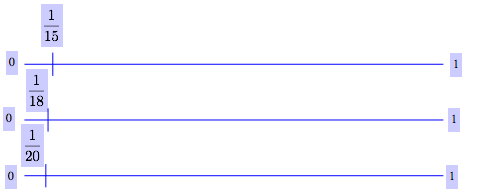### Home > MC1 > Chapter 10 > Lesson 10.2.3 > Problem10-99

10-99.

Multiple Choice: Which of the following fractions is closest to $1$? Explain how you can tell.

1. $\frac { 19 } { 18 }$

1. $\frac { 19 } { 20 }$

1. $1\frac { 1 } { 15 }$

1. $\frac { 17 } { 18 }$

Fractions are show below to help you visualize. Use the size of each fraction to determine which option is closest to $1$.